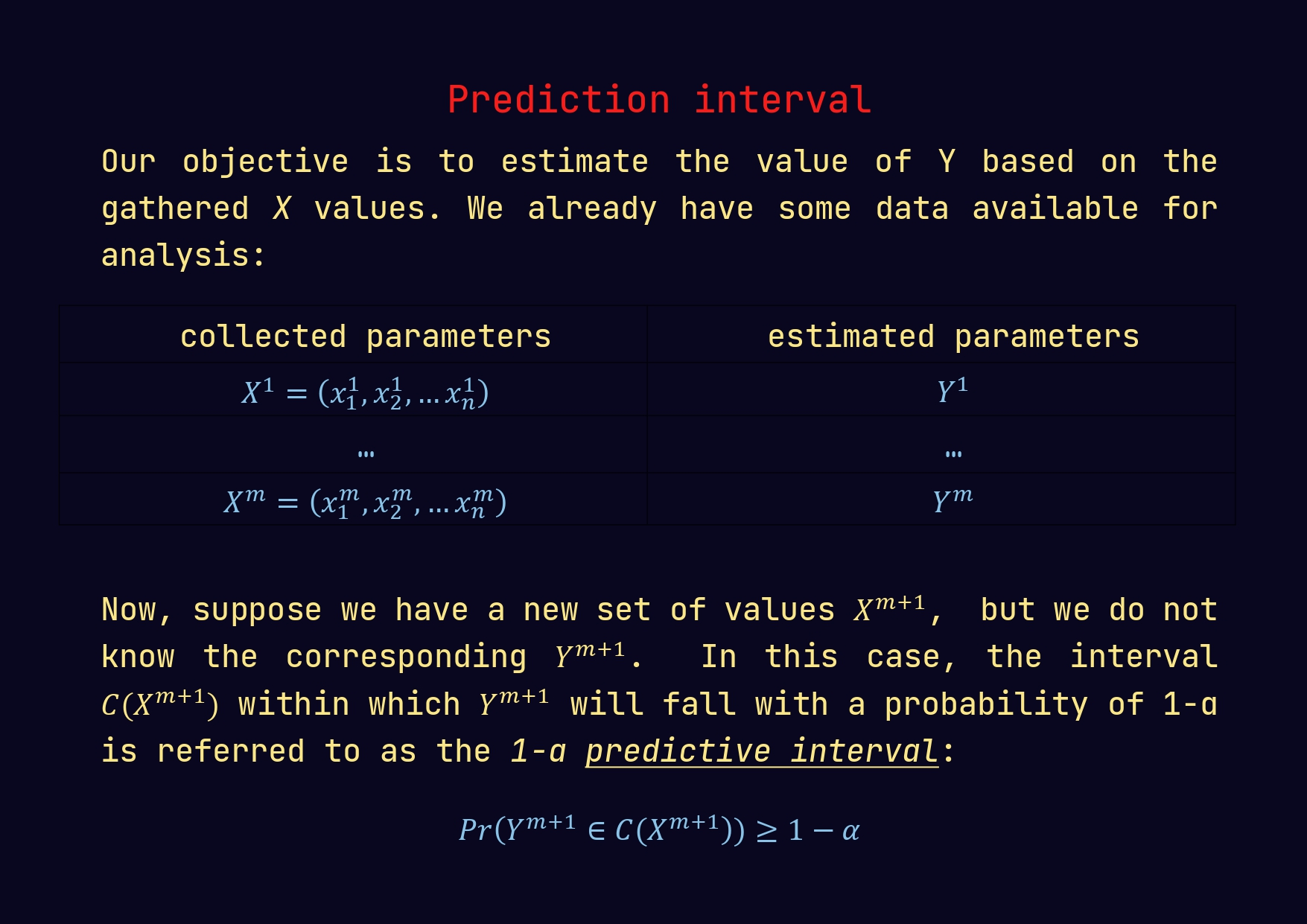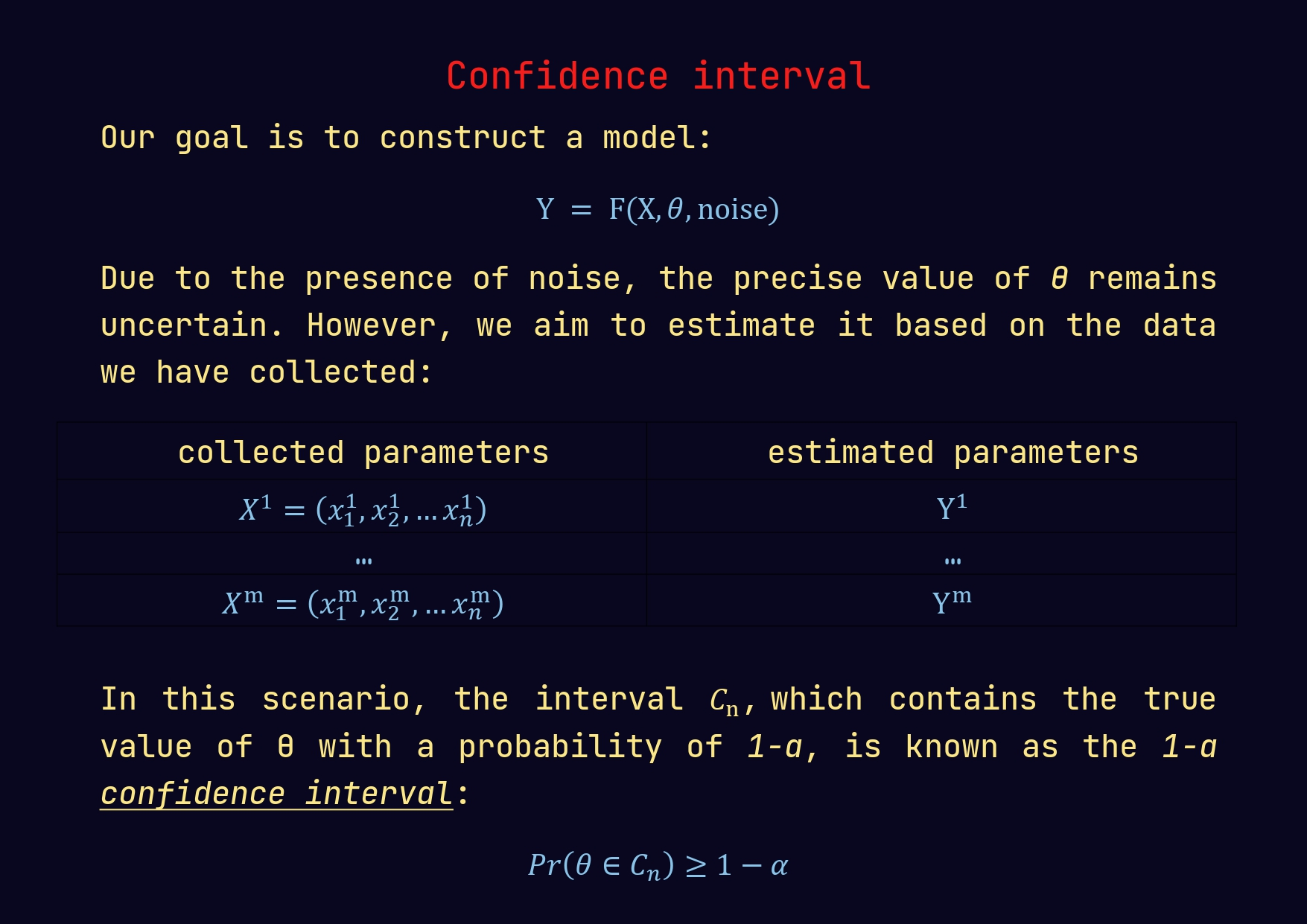Let’s dive into the world of linear regression and explore the concepts of confidence intervals for model coefficients and predictive intervals for dependent variable. 🎯

Coefficients represent the relationships between predictor variables and the response variable. However, real-world data is often noisy, leading to varying coefficient estimates from one dataset to another. That’s where confidence intervals come in! These intervals provide a safety net, quantifying the uncertainty around our coefficient estimates.

🎯 Why Do We Need Confidence Intervals?
Uncertainty Awareness: They help us understand the degree of uncertainty associated with our coefficient estimates, acknowledging that our sample might not perfectly represent the entire population.

Statistical Significance: Confidence intervals are instrumental in determining if a coefficient is statistically significant. If the interval doesn’t include zero, the coefficient likely has a significant effect on the response variable.

Comparisons and Decision Making: They allow us to compare coefficients across different models or datasets. Non-overlapping intervals suggest significant differences.

Drawing Inferences: Confidence intervals help us draw more robust conclusions. We can confidently say that our coefficient is within the interval with a certain probability (typically 95%).

🔍 Predictive Intervals: Going a Step Further
Apart from confidence intervals, predictive intervals are another crucial concept in linear regression.

🎯 What Are Predictive Intervals?
Predictive intervals take uncertainty a step further. They provide a range of values for the actual response variable at a given predictor variable value, estimating where the true response value is likely to fall for a new observation.

Individual Prediction: Unlike confidence intervals, predictive intervals estimate where an individual response value might lie for a specific predictor value.
Accounting for Variability: Predictive intervals account for both the uncertainty in coefficient estimates and the inherent variability in the response variable, making them wider than confidence intervals.

🔍 Interpreting Predictive Intervals:
A typical interpretation of a 95% predictive interval might be:
“We are 95% confident that the true response value lies within the range [lower_bound, upper_bound] for a given predictor value.”

📈 Embrace the Power of Intervals
In conclusion, confidence intervals for model coefficients and predictive intervals for the dependent variable are essential tools for understanding uncertainty and making informed predictions. They help us assess statistical significance, draw meaningful insights, and make decisions based on our data. So next time you interpret a linear regression model, don’t forget to check those confidence and predictive intervals! 📊